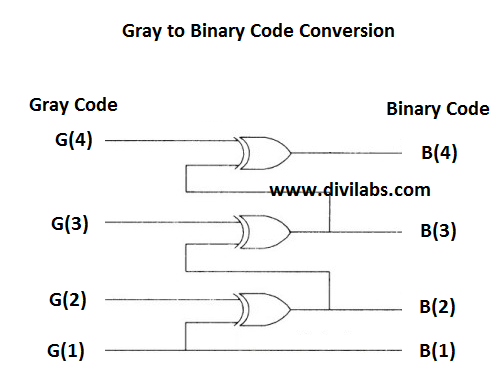# Gray To Binary Code Conversion in MATLAB without using MATLAB's Inbuilt Function

In the previous post, Binary To Gray Code Conversion in MATLAB without using MATLAB's Inbuilt Function, we have converted Binary code to Gray code.
Now its time for performing the reverse process.
The process of converting a Gray code to a Binary code also requiring XORing, but with the resultant bits with the input bits, rather than a pair or input bits that we have done previously while converting a Binary code to Gray one.

This process can be illustrated as:GATE Level Logic Diagram of Gray to Binary Code ConversionMATLAB: How the Implementation of Gray Code To Binary Code conversion is done
Suppose we had originally a binary bit stream of 30 bits,
a =
0     1     0     0     1     1     1     0     1     1     1     0     1     1     0     0     1     1     0     1     1     0     0     0     0     0     0     1     0     1

After Gray Coding the Bit Stream we got like this,
g =
0     1     1     0     1     0     0     1     1     0     0     1     1     0     1     0     1     0     1     1     0     1     0     0     0     0     0     1     1     1

So to check our code is working well or not we will give 'g' as an input to recover the original binary bit sequence 'a'. You can find the opposite here, Binary To Gray Code Conversion in MATLAB without using MATLAB's Inbuilt Function.

MATLAB code for Conversion of Gray Code to Binary Code is:
%if you want to use your own sequence just declare or gather the variable 'g' before the start of following lines
x(:,1) = g(:,1); %copying the 1st gray bit as it is, to the resultant vector

for i = 2:size(g,2),
x(:,i) = xor( x(:,i-1), g(:,i) );
%XORing the following bits as per the procedure
end

The resultant value of x is,
x =
0     1     0     0     1     1     1     0     1     1     1     0     1     1     0     0     1     1     0     1     1     0     0     0     0     0     0     1     0     1

On comparing with the original Binary Sequence 'a' we find that 'x' is same to it. So our code is converting the gray code to binary code very well.